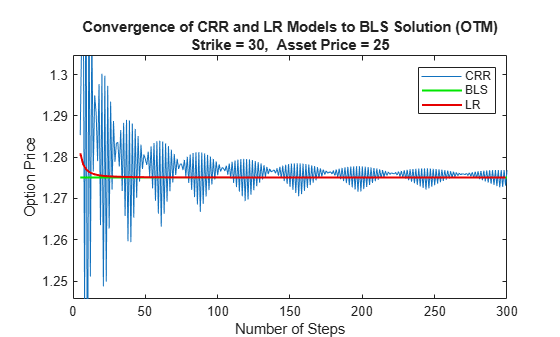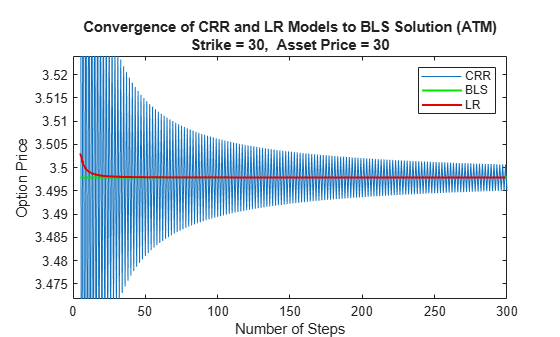# Pricing European Call Options Using Different Equity Models

This example illustrates how the Financial Instruments Toolbox™ is used to price European vanilla call options using different equity models.

The example compares call option prices using the Cox-Ross-Rubinstein model, the Leisen-Reimer model and the Black-Scholes closed formula.

### Define the Call Instrument

Consider a European call option, with an exercise price of \$30 on January 1, 2010. The option expires on Sep 1, 2010. Assume that the underlying stock provides no dividends. The stock is trading at \$25 and has a volatility of 35% per annum. The annualized continuously compounded risk-free rate is 1.11% per annum.

```% Option Settle = 'Jan-01-2010'; Maturity = 'Sep-01-2010'; Strike = 30; OptSpec = 'call'; % Stock AssetPrice = 25; Sigma = .35;```

### Create the Interest Rate Term Structure

```StartDates = '01 Jan 2010'; EndDates = '01 Jan 2013'; Rates = 0.0111; ValuationDate = '01 Jan 2010'; Compounding = -1; RateSpec = intenvset('Compounding',Compounding,'StartDates', StartDates,... 'EndDates', EndDates, 'Rates', Rates,'ValuationDate', ValuationDate);```

### Create the Stock Structure

Suppose we want to create two scenarios. The first one assumes that `AssetPrice` is currently \$25, the option is out of the money (OTM). The second scenario assumes that the option is at the money (ATM), and therefore `AssetPriceATM` = `30`.

```AssetPriceATM = 30; StockSpec = stockspec(Sigma, AssetPrice); StockSpecATM = stockspec(Sigma, AssetPriceATM);```

### Price the Options Using the Black-Scholes Closed Formula

Use the function `optstockbybls` in the Financial Instruments Toolbox to compute the price of the European call options.

```% Price the option with AssetPrice = 25 PriceBLS = optstockbybls(RateSpec, StockSpec, Settle, Maturity, OptSpec, Strike); % Price the option with AssetPrice = 30 PriceBLSATM = optstockbybls(RateSpec, StockSpecATM, Settle, Maturity, OptSpec, Strike);```

### Build the Cox-Ross-Rubinstein Tree

```% Create the time specification of the tree NumPeriods = 15; CRRTimeSpec = crrtimespec(ValuationDate, Maturity, NumPeriods); % Build the tree CRRTree = crrtree(StockSpec, RateSpec, CRRTimeSpec); CRRTreeATM = crrtree(StockSpecATM, RateSpec, CRRTimeSpec);```

### Build the Leisen-Reimer Tree

```% Create the time specification of the tree LRTimeSpec = lrtimespec(ValuationDate, Maturity, NumPeriods); % Use the default method 'PP1' (Peizer-Pratt method 1 inversion)to build % the tree LRTree = lrtree(StockSpec, RateSpec, LRTimeSpec, Strike); LRTreeATM = lrtree(StockSpecATM, RateSpec, LRTimeSpec, Strike);```

### Price the Options Using the Cox-Ross-Rubinstein (CRR) Model

```PriceCRR = optstockbycrr(CRRTree, OptSpec, Strike, Settle, Maturity); PriceCRRATM = optstockbycrr(CRRTreeATM, OptSpec, Strike, Settle, Maturity);```

### Price the Options Using the Leisen-Reimer (LR) Model

```PriceLR = optstockbylr(LRTree, OptSpec, Strike, Settle, Maturity); PriceLRATM = optstockbylr(LRTreeATM, OptSpec, Strike, Settle, Maturity);```

### Compare BLS, CRR and LR Results

```sprintf('PriceBLS: \t%f\nPriceCRR: \t%f\nPriceLR:\t%f\n', PriceBLS, ... PriceCRR, PriceLR)```
```ans = 'PriceBLS: 1.275075 PriceCRR: 1.294979 PriceLR: 1.275838 ' ```
```sprintf('\t== ATM ==\nPriceBLS ATM: \t%f\nPriceCRR ATM: \t%f\nPriceLR ATM:\t%f\n', PriceBLSATM, ... PriceCRRATM, PriceLRATM)```
```ans = ' == ATM == PriceBLS ATM: 3.497891 PriceCRR ATM: 3.553938 PriceLR ATM: 3.498571 ' ```

### Convergence of CRR and LR Models to a BLS Solution

The following tables compare call option prices using the CRR and LR models against the results obtained with the Black-Scholes formula.

While the CRR binomial model and the Black-Scholes model converge as the number of time steps gets large and the length of each step gets small, this convergence, except for at the money options, is anything but smooth or uniform.

The tables below show that the Leisen-Reimer model reduces the size of the error with even as few steps of 45.

` Strike = 30, Asset Price = 30 `

` -------------------------------------`

` #Steps LR CRR `

` 15 3.4986 3.5539`

` 25 3.4981 3.5314`

` 45 3.4980 3.5165`

` 65 3.4979 3.5108`

` 85 3.4979 3.5077`

` 105 3.4979 3.5058`

` 201 3.4979 3.5020`

` 501 3.4979 3.4996`

` 999 3.4979 3.4987`

` Strike = 30, Asset Price = 25 `

` -------------------------------------`

` #Steps LR CRR `

` 15 1.2758 1.2950`

` 25 1.2754 1.2627`

` 45 1.2751 1.2851`

` 65 1.2751 1.2692`

` 85 1.2751 1.2812`

` 105 1.2751 1.2766`

` 201 1.2751 1.2723`

` 501 1.2751 1.2759`

` 999 1.2751 1.2756`

### Analyze the Effect of the Number of Periods on the Price of the Options

The following graphs show how convergence changes as the number of steps in the binomial calculation increases, as well as, the impact on convergence to changes to the stock price. Observe that the Leisen-Reimer model removes the oscillation and produces estimates close to the Black-Scholes model using only a small number of steps.

```NPoints = 300; % Cox-Ross-Rubinstein NumPeriodCRR = 5 : 1 : NPoints; NbStepCRR = length(NumPeriodCRR); PriceCRR = nan(NbStepCRR, 1); PriceCRRATM = PriceCRR; for i = 1 : NbStepCRR CRRTimeSpec = crrtimespec(ValuationDate, Maturity, NumPeriodCRR(i)); CRRT = crrtree(StockSpec, RateSpec, CRRTimeSpec); PriceCRR(i) = optstockbycrr(CRRT, OptSpec, Strike,ValuationDate, Maturity) ; CRRTATM = crrtree(StockSpecATM, RateSpec, CRRTimeSpec); PriceCRRATM(i) = optstockbycrr(CRRTATM, OptSpec, Strike,ValuationDate, Maturity) ; end % Now with Leisen-Reimer NumPeriodLR = 5 : 2 : NPoints; NbStepLR = length(NumPeriodLR); PriceLR = nan(NbStepLR, 1); PriceLRATM = PriceLR; for i = 1 : NbStepLR LRTimeSpec = lrtimespec(ValuationDate, Maturity, NumPeriodLR(i)); LRT = lrtree(StockSpec, RateSpec, LRTimeSpec, Strike); PriceLR(i) = optstockbylr(LRT, OptSpec, Strike,ValuationDate, Maturity) ; LRTATM = lrtree(StockSpecATM, RateSpec, LRTimeSpec, Strike); PriceLRATM(i) = optstockbylr(LRTATM, OptSpec, Strike,ValuationDate, Maturity) ; end```

First scenario: Out of the Money call option

```% For Cox-Ross-Rubinstein plot(NumPeriodCRR, PriceCRR); hold on; plot(NumPeriodCRR, PriceBLS*ones(NbStepCRR,1),'Color',[0 0.9 0], 'linewidth', 1.5); % For Leisen-Reimer plot(NumPeriodLR, PriceLR, 'Color',[0.9 0 0], 'linewidth', 1.5); % Concentrate in the area of interest by clipping on the Y axis at 5x the % LR Price: YLimDelta = 5*abs(PriceLR(1) - PriceBLS); ax = gca; ax.YLim = [PriceBLS-YLimDelta PriceBLS+YLimDelta]; % Annotate Plot titleString = sprintf('\nConvergence of CRR and LR models to a BLS Solution (OTM)\nStrike = %d, Asset Price = %d', Strike , AssetPrice); title(titleString) ylabel('Option Price') xlabel('Number of Steps') legend('CRR', 'BLS', 'LR', 'Location', 'NorthEast')```Second scenario: At the Money call option

```% For Cox-Ross-Rubinstein figure; plot(NumPeriodCRR, PriceCRRATM); hold on; plot(NumPeriodCRR, PriceBLSATM*ones(NbStepCRR,1),'Color',[0 0.9 0], 'linewidth', 1.5); % For Leisen-Reimer plot(NumPeriodLR, PriceLRATM, 'Color',[0.9 0 0], 'linewidth', 1.5); % Concentrate in the area of interest by clipping on the Y axis at 5x the % LR Price: YLimDelta = 5*abs(PriceLRATM(1) - PriceBLSATM); ax = gca; ax.YLim = [PriceBLSATM-YLimDelta PriceBLSATM+YLimDelta]; % Annotate Plot titleString = sprintf('\nConvergence of CRR and LR models to a BLS Solution (ATM)\nStrike = %d, Asset Price = %d', Strike , AssetPriceATM); title(titleString) ylabel('Option Price') xlabel('Number of Steps') legend('CRR', 'BLS', 'LR', 'Location', 'NorthEast')```